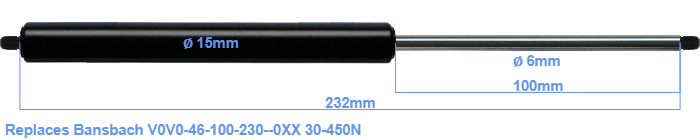# Replacement for Bansbach V0V0-46-100-230–0XX 30-450N

\$26.73

Replacement gas spring for the Bansbach V0V0-46-100-230--0XX 30-450 Newton. Thread: M5. Brand: HAHN, a Stabilus company.
 Force Choose an option30 Newton40 Newton50 Newton60 Newton80 Newton100 Newton120 Newton140 Newton150 Newton160 Newton180 Newton200 Newton220 Newton240 Newton250 Newton260 Newton280 Newton300 Newton320 Newton340 Newton350 Newton360 Newton380 Newton400 Newton420 Newton440 Newton450 NewtonClearThe diameter of the cylinder of this gas spring is 15 millimeters. The rod has a diameter of 6 mm. The rod (the chrome part) is 100 mm long. The total length of this replacement is exactly 232 mm. Attention: this is the length between the centers of the mounting parts. Without the mounting parts this gas spring is 232 mm long (thread to thread). The force of this replacement gas spring is 30-450 Newton. This is not an official Bansbach gas spring, but a so called replacement. This replacement gas spring is a HAHN gas spring. The gas spring can be used without problems as a replacement, because the dimensions and force equal.